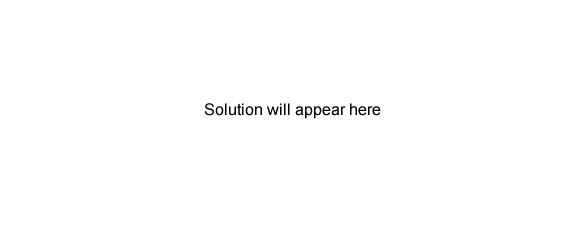General exercises (2005 VCE) 0.10 mole of C4H9OH reacts completely with molecular oxygen, O2. The number of mole of oxygen molecules used is A. 0.50 B. 0.55 C. 0.60 D. 0.65 Solution 50.00 mL of a 0.020 M solution of Ba(OH)2 is added to 50.00 mL of a 0.060 M solution of HNO3 . The hydrogen ion concentration in the resultant solution, in mole per litre, is A. 0.010 B. 0.020 C. 0.030 D. 0.040 Solution Sodium hydride (NaH) reacts with water as follows. NaH(s) + H2O(l) → Na+(aq) + OH-(aq) + H2(g) This reaction should be classified as A. acid-base but not redox. B. redox but not acid-base. C. both acid-base and redox. D. neither redox nor acid-base. Solution Hydrogen and chlorine react according to the equation H2(g) + Cl2(g) → 2HCl(g) 3 mole of H2 and 2 mole of Cl2 are placed in a vessel and sealed. When reaction is complete the vessel will contain A. 5 mole of HCl B. 6 mole of HCl and 1 mole of Cl2 C. 4 mole of HCl and 1 mole of Cl2 D. 4 mole of HCl and 1 mole of H2 Solution An organic compound is known to contain only carbon, hydrogen and oxygen. The compound contains, by mass, 39.1% of carbon and 8.7% of hydrogen. The number of carbon atoms in the empirical formula is A. 1 B. 2 C. 3 D. 4 SolutionA solution of sodium hydroxide (NaOH) has a pH of 10. 10 mL of this solution is mixed with 990 mL of water. The pH of the diluted solution is closest to A. 8 B. 9 C. 11 D. 12 Solution 20.0 mL of 0.10 M hydrochloric acid (HCl) reacts with 20.0 mL of 0.30 M potassium hydroxide (KOH) solution. The concentration of potassium ions in the resultant solution, in mole per litre, is A. 0.10 B. 0.15 C. 0.20 D. 0.30 Solution A 100 mL sample of helium exerts a pressure of 1 atm at 10°C. The volume of the container is reduced to 50 mL and then the temperature is increased to 20°C. The pressure now exerted by the helium, in atmosphere, is closest to A. 0.5 B. 1 C. 2 D. 4 Solution